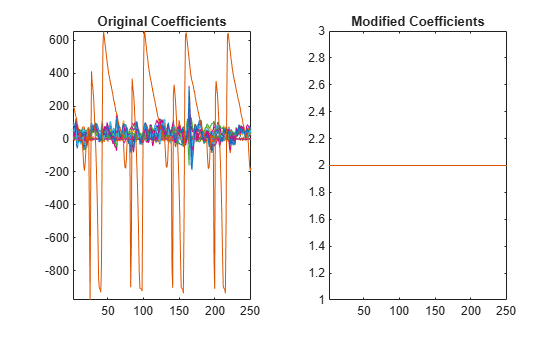# chgwdeccfs

Change multisignal 1-D decomposition coefficients

## Syntax

```DEC = chgwdeccfs(DEC,'ca',COEFS) DEC = chgwdeccfs(DEC,'cd',COEFS,LEV) DEC = chgwdeccfs(DEC,'all',CA,CD) DEC = chgwdeccfs(DEC,'all',V) DEC = chgwdeccfs(...,IDXSIG) ```

## Description

`DEC = chgwdeccfs(DEC,'ca',COEFS)` replaces the approximation coefficients at level `DEC.level` with those contained in the matrix `COEFS`. If `COEFS` is a single value `V`, all coefficients are replaced by `V`.

`DEC = chgwdeccfs(DEC,'cd',COEFS,LEV)` replaces the detail coefficients at level `LEV` with those contained in the matrix `COEFS`. If `COEFS` is a single value `V`, then `LEV` can be a vector of levels and all the coefficients that belong to these levels are replaced by `V`. `LEV` must be such that `1 `` LEV `` DEC.level`

`DEC = chgwdeccfs(DEC,'all',CA,CD)` replaces all the approximation and detail coefficients. `CA` must be a matrix and `CD` must be a cell array of length `DEC.level`.

If `COEFS` (or `CA` or `CD`) is a single number, then it replaces all the related coefficients. Otherwise, `COEFS` (or `CA`, or `CD`) must be a matrix of appropriate size.

For a real value `V`, ```DEC = chgwdeccfs(DEC,'all',V)``` replaces all the coefficients by `V`.

`DEC = chgwdeccfs(...,IDXSIG)` replaces the coefficients for the signals whose indices are given by the vector `IDXSIG`. If the initial data are stored row-wise or column-wise in a matrix `X`, then `IDXSIG` contains the row or column indices, respectively, of the data.

## Examples

collapse all

Load the 23 channel EEG data `Espiga3` . The channels are arranged column-wise. The data is sampled at 200 Hz.

`load Espiga3`

Perform a decomposition at level 2 using the `db2` wavelet.

`dec = mdwtdec('c',Espiga3,2,'db2')`
```dec = struct with fields: dirDec: 'c' level: 2 wname: 'db2' dwtFilters: [1x1 struct] dwtEXTM: 'sym' dwtShift: 0 dataSize: [995 23] ca: [251x23 double] cd: {[499x23 double] [251x23 double]} ```

Change the coefficients of details at level 1. Replace all the values by 0.

`decBis = chgwdeccfs(dec,'cd',0,1);`

Change the coefficients of details at level 1 and level 2 for signals 11 to 15. Replace all values by 0.

`decTer = chgwdeccfs(dec,'cd',0,1:2,11:15);`

Compare the original and new coefficients for details at level 1 for signals 11 to 15.

```plot(dec.cd{1}(:,11:15),'b') hold on plot(decTer.cd{1}(:,11:15),'r') legend('Original','Changed')```Mesa, Hector. “Adapted Wavelets for Pattern Detection.” In Progress in Pattern Recognition, Image Analysis and Applications, edited by Alberto Sanfeliu and Manuel Lazo Cortés, 3773:933–44. Berlin, Heidelberg: Springer Berlin Heidelberg, 2005. https://doi.org/10.1007/11578079_96.

## Support